# NCERT Solutions class 7 Maths Chapter-3 Exercise 3.3

NCERT Solutions Class-7 Maths chapter-3 data handling Exercise-3.3 is prepared by academic team of Physics Wallah all the questions of NCERT text book are solved step by step with proper and detail solutions explaining each and every questions. For More and additional questions of CBSE class 7 mathsyou can go to class 7 maths sections.NCERT class 7 Maths Solutionsis the best way to enhanced your mathematics skill. And Physics Wallah practice worksheet & question bank will help you a lot .

## Question 1:

Use the bar graph (see the given figure) to answer the following questions.

(a) Which is the most popular pet?

(b) How many students have dog as a pet?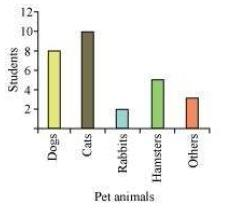(a) Here,

According to the question,

We have to find the most popular pet among them.

Now,

Here,

The bar graph represents the pets owned by the students of class seven.

And,

We can see that,

The bar of the cat is the tallest

Hence,

Cat is the most popular pet.

Since the bar representing cats is the tallest, cat is the most popular pet.

(b) Here,

According to the question,

We have to find the number of students who have dog as a pet.

Now,

Here,

We know that,

The bar graph represents the pets owned by the students of class seven.

And,

We can see that,

The bar of the dog is till 8

Hence,

8 students have dog as their pet.

The number of students having dog as a pet are 8.

Question 2:

Read the bar graph (see the given figure) which shows the number of books sold by a bookstore during five consecutive years and answer the questions that follow: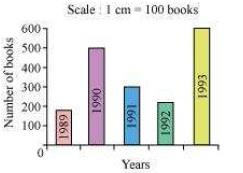(i) About how many books were sold in 1989? 1990? 1992?

(ii) In which year were about 475 books sold? About 225 books sold?

(iii) In which years were fewer than 250 books sold?

(iv) Can you explain how you would estimate the number of books sold in 1989?

(i) Here,

We can say that,

While observing the graph,

The total books sold in the year 1989, 1990 and 1992 are as follows:

Number of books sold in 1989 = 175

And,

Number of books sold in 1992 = 225

And,

Number of books sold in 1990 = 475

In 1989, 175 books were sold. In 1990, 475 books were sold. In 1992, 225 books were sold.

(ii) Here,

We have to find,

The year in which the following number of books were sold:

475

And,

225

Hence,

While observing the graph,

We can say that,

475 books were sold in the year 1990

And,

225 books were sold in the year 1992

From the graph, it can be concluded that 475 books were sold in the year 1990 and 225 books were sold in the year 1992.

(iii)Here,

We have to find,

The years in which the books sold were less than 250 books

Hence,

While observing the graph,

We can conclude that,

The years in which the total number of books sold was less than 250 are as follows:

The year 1989 And The year 1992

From the graph, it can be concluded that in the years 1989 and 1992, the number of books sold were less than 250.

(iv) From the graph, it can be concluded that the number of books sold in the year 1989 is about 1 and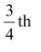part of 1 cm.

We know that the scale is taken as 1 cm = 100 books.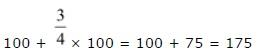Therefore, about 175 books were sold in the year 1989.

Question 3:

Number of children in six different classes are given below. Represent the data on a bar graph.

 Class Fifth Sixth Seventh Eighth Ninth Tenth Number of children 135 120 95 100 90 80

(a) How would you choose a scale?

(i) Which class has the maximum number of children? And the minimum?

(ii) Find the ratio of students of class sixth to the students of class eight.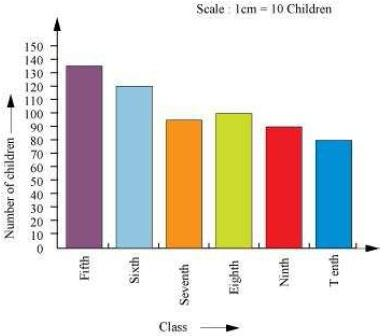(a) We will choose a scale as 1 unit = 10 children because we can represent a more clear difference between the number of students of class 7th and that of class 9th by this scale.

(b) (i) Since the bar representing the number of children for class fifth is the tallest, there are maximum number of children in class fifth. Similarly, since the bar representing the number of children for class tenth is the smallest, there are minimum number of children in class tenth.

(ii) The number of students in class sixth is 120 and the number of students in class eighth is 100.

Therefore, the ratio between the number of students of class sixth and the number of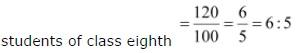Question 4:

The performance of students in 1st Term and 2nd Term is given. Draw a double bar graph choosing appropriate scale and answer the following:

 Subject English Hindi Maths Science S.science 1st Term (M.M. 100) 67 72 88 81 73 2nd Term (M.M. 100) 70 65 95 85 75

(i) In which subject, has the child improved his performance the most?

(ii) In which subject is the improvement the least?

(iii) Has the performance gone down in any subject?

A double bar graph for the given data is as follows.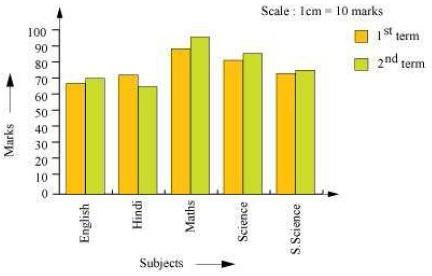(i) Here,

We can see that,

Math had a maximum increase in marks.

Hence,

It can be concluded that,

The child had improved his performance the most in Maths.

There was a maximum increase in the marks obtained in Maths. Therefore, the child has improved his performance the most in Maths.

(ii) Here,

We can see that,

S. science had the least increase in marks.

Hence,

It can be concluded that,

The child had improved his performance the least in S. science.

From the graph, it can be concluded that the improvement was the least in S. Science.

(iii) Here,

We can observe that,

From the given graph,

Hindi has a decrement in marks in the second term

Hence,

It can be concluded that,

The child’s performance has gone down in Hindi.

From the graph, it can be observed that the performance in Hindi has gone down.

Question 5:

Consider this data collected from a survey of a colony.

 Favourite sport Cricket Basket Ball Swimming Hockey Athletics Watching 1240 470 510 430 250 Participating 620 320 320 250 105

(i) Draw a double bar graph choosing an appropriate scale. What do you infer from the bar graph?

(ii) Which sport is most popular?

(iii) Which is more preferred, watching or participating in sports?

(i)A double bar graph for the given data is as follows.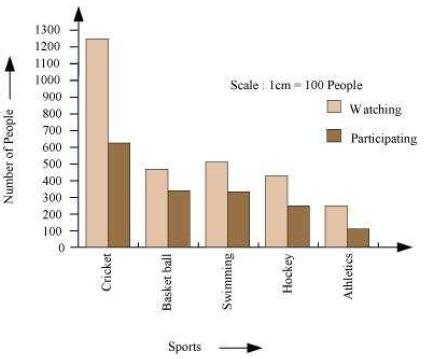(i) Draw a double bar graph choosing an appropriate scale. What do you infer from the bar graph?

(ii) Which sport is most popular?

(iii) Which is more preferred, watching or participating in sports?

(i)A double bar graph for the given data is as follows.The double bar graph represents the number of people who like watching and participating in different sports. It can be observed that most of the people like watching and participating in cricket while the least number of people like watching and participating in athletics.

(ii) From the bar graph, it can be observed that the bar representing the number of people who like watching and participating in cricket is the tallest among all the bars. Hence, cricket is the most popular sport.

(iii) The bars representing watching sport are longer than the bars representing participating in sport. Hence, watching different types of sports is more preferred than participating in the sports.

Question 6:

Take the data giving the minimum and the maximum temperature of various cities given in the following table:

Temperatures of the cities as on 20.6.2006

 City Max. Min. Ahmedabad 38 ºC 29 ºC Amritsar 37 ºC 26 ºC Banglore 28 ºC 21 ºC Chennai 36 ºC 27 ºC Delhi 38 ºC 28 ºC Jaipur 39 ºC 29 ºC Jammu 41 ºC 26 ºC Mumbai 32 ºC 27 ºC

Plot a double bar graph using the data and answer the following:

(i) Which city has the largest difference in the minimum and maximum temperature on the given date?

(ii) Which is the hottest city and which is the coldest city?

(iii) Name two cities where maximum temperature of one was less than the minimum temperature of the other.

(iv) Name the city which has the least difference between its minimum and the maximum temperature.

A double bar graph for the given data is constructed as follows.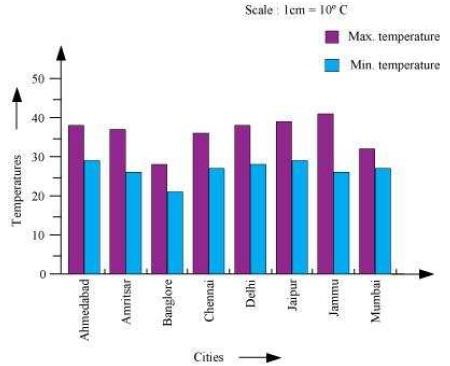(i) From the graph, it can be concluded that Jammu has the largest difference in its minimum and maximum temperatures on 20.6.2006.

(ii) From the graph, it can be concluded that Jammu is the hottest city and Bangalore is the coldest city.

(iii) Bangalore and Jaipur, Bangalore and Ahmedabad

For Bangalore, the maximum temperature was 28ºC, while minimum temperature of both cities, Ahmedabad and Jaipur, was 29ºC.

(iv) From the graph, it can be concluded that the city which has the least difference between its minimum and maximum temperatures is Mumbai.